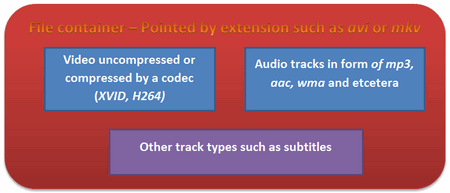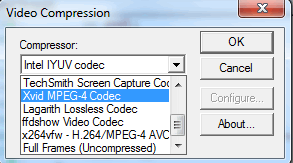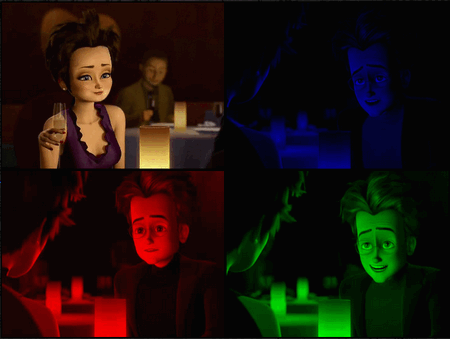# 用OpenCV创建视频¶

## 目标¶

• 如何用OpenCV创建一个视频文件
• 用OpenCV能创建什么样的视频文件
• 如何释放视频文件当中的某个颜色通道

• 第一个参数指向你需要操作的视频文件。
• 第二个参数可以是如下的几个字母之一：R G B。用来指定你需要释放哪一个通道。
• 最后一个参数是Y(Yes)或者N(No). 如果你选择N, 就直接使用视频输入格式来创建输出文件，否则就会弹出一个对话框来让你选择编码器。

```video-write.exe video/Megamind.avi R Y
```

## 源代码¶

 ``` 1 2 3 4 5 6 7 8 9 10 11 12 13 14 15 16 17 18 19 20 21 22 23 24 25 26 27 28 29 30 31 32 33 34 35 36 37 38 39 40 41 42 43 44 45 46 47 48 49 50 51 52 53 54 55 56 57 58 59 60 61 62 63 64 65 66 67 68 69 70 71 72 73 74 75 76 77 78 79 80 81 82 83 84``` ```#include // for standard I/O #include // for strings #include // Basic OpenCV structures (cv::Mat) #include // Video write using namespace std; using namespace cv; int main(int argc, char *argv[], char *window_name) { if (argc != 4) { cout << "Not enough parameters" << endl; return -1; } const string source = argv; // the source file name const bool askOutputType = argv =='Y'; // If false it will use the inputs codec type VideoCapture inputVideo(source); // Open input if ( !inputVideo.isOpened()) { cout << "Could not open the input video." << source << endl; return -1; } string::size_type pAt = source.find_last_of('.'); // Find extension point const string NAME = source.substr(0, pAt) + argv + ".avi"; // Form the new name with container int ex = static_cast(inputVideo.get(CV_CAP_PROP_FOURCC)); // Get Codec Type- Int form // Transform from int to char via Bitwise operators char EXT[] = {ex & 0XFF , (ex & 0XFF00) >> 8,(ex & 0XFF0000) >> 16,(ex & 0XFF000000) >> 24, 0}; Size S = Size((int) inputVideo.get(CV_CAP_PROP_FRAME_WIDTH), //Acquire input size (int) inputVideo.get(CV_CAP_PROP_FRAME_HEIGHT)); VideoWriter outputVideo; // Open the output if (askOutputType) outputVideo.open(NAME , ex=-1, inputVideo.get(CV_CAP_PROP_FPS),S, true); else outputVideo.open(NAME , ex, inputVideo.get(CV_CAP_PROP_FPS),S, true); if (!outputVideo.isOpened()) { cout << "Could not open the output video for write: " << source << endl; return -1; } union { int v; char c;} uEx ; uEx.v = ex; // From Int to char via union uEx.c='\0'; cout << "Input frame resolution: Width=" << S.width << " Height=" << S.height << " of nr#: " << inputVideo.get(CV_CAP_PROP_FRAME_COUNT) << endl; cout << "Input codec type: " << EXT << endl; int channel = 2; // Select the channel to save switch(argv) { case 'R' : {channel = 2; break;} case 'G' : {channel = 1; break;} case 'B' : {channel = 0; break;} } Mat src,res; vector spl; while( true) //Show the image captured in the window and repeat { inputVideo >> src; // read if( src.empty()) break; // check if at end split(src, spl); // process - extract only the correct channel for( int i =0; i < 3; ++i) if (i != channel) spl[i] = Mat::zeros(S, spl.type()); merge(spl, res); //outputVideo.write(res); //save or outputVideo << res; } cout << "Finished writing" << endl; return 0; } ```

## 视频文件的结构¶## VideoWriter 类¶

1. 输出的文件名中包含了容器的类型，当然在现在仅仅支持 avi 格式。在这个例子中我们会使用输入文件名+通道名+avi来创建输出文件名。

```const string source      = argv;            // 原视频文件名
string::size_type pAt = source.find_last_of('.');   // 找到扩展名的位置
const string NAME = source.substr(0, pAt) + argv + ".avi";   // 创建新的视频文件名
```
2. 然后决定使用的编解码器，现在所有的视频编解码器都使用最多四个字节的名称来标识，例如 XVID, DIVX, 和 H264 等。这个被称作FourCC（four character code）。你可以通过 get 函数来向视频询问这个编码， get 函数会返回一个double数，仅仅是因为double包含了64位数据而已，由于FourCC编码只占据了其中低位的4个字节，所以可以直接通过强制转换成int型来扔掉高位的四个字节。

```VideoCapture inputVideo(source);                                   // 打开视频输入
int ex = static_cast<int>(inputVideo.get(CV_CAP_PROP_FOURCC));     // 得到编码器的int表达式
```

OpenCV内部使用这个int数来当作第二个参数，这里会使用两种方法来将这个整型数转换为字符串：位操作符和联合体。前者可以用&操作符并进行移位操作，以便从int里面释放出字符：

```char EXT[] = {ex & 0XFF , (ex & 0XFF00) >> 8,(ex & 0XFF0000) >> 16,(ex & 0XFF000000) >> 24, 0};
```

```union { int v; char c;} uEx ;
uEx.v = ex;                              // 通过联合体来分解字符串
uEx.c='\0';

反过来，当你需要修改视频的格式时，你都需要修改FourCC码，而更改ForCC的时候都要做逆转换来指定新类型。如果你已经知道这个FourCC编码的具体字符的话，可以直接使用 *CV_FOURCC* 宏来构建这个int数:```1. 输出视频的帧率，也就是每秒需要绘制的图像数，在这里我让输出视频的帧率与输入视频相同，输入视频的帧率可以由 get 函数来获得。
2. 输出视频的尺寸，在这里我同样保持和输入视频一样的大小，这个大小同样也可以由 get 函数来获得。
3. 最后一个参数是一个可选参数。默认下它是true，来表示输出的视频是彩色的（所以你需要传给它三通道的图像），如果想创建一个灰度视频就传入false。

```VideoWriter outputVideo;
Size S = Size((int) inputVideo.get(CV_CAP_PROP_FRAME_WIDTH),    //获取输入尺寸
(int) inputVideo.get(CV_CAP_PROP_FRAME_HEIGHT));

outputVideo.open(NAME , ex, inputVideo.get(CV_CAP_PROP_FPS),S, true);
```

```outputVideo.write(res);  //或者
outputVideo << res;
```

```split(src, spl);                 // 分离三个通道
for( int i =0; i < 3; ++i)
if (i != channel)
spl[i] = Mat::zeros(S, spl.type());         //创建相同大小的黑色图像
merge(spl, res);             //重新合并
```## 翻译者¶

nahcooo@ OpenCV中文网站 <nahcooo@gmail.com>